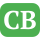CppBuzz.com

# Need to save a single line from delete on some special condition

```I have written a perl code which is able to search and delete a line from a file (IN_FILE) which contains "max_transition",when "direction : output" condition will satisfy. After deleting the line the code writes the modified file into a different location.

Now I need to delete the line "max_transition" when the "direction : output" condition will satisfy as well as where it will find word "timing" in that particular pin group.

IN_FILE, has output pin "HIZIBI_79", HIZIBI_78 and HIZIBI. After running the script "max_transition" line should not be deleted from pin "HIZIBI_79", HIZIBI_78", because this pin group do not have "timing". Only "max_transition" line would be deleted from pin "HIZIBI", it has a "timing" group.

Any idea how to implement it?

Output file should be look like OUT_FILE

PERL CODE
use warnings;
use strict;
my \$inputfile = \$ARGV; # input lib FILE

if (\$#ARGV!=0)
{
print "USAGE :: perl max_tran_update.pl <<LIB_FILE>>  \n\n" ;
exit(1);
}

my \$cmd = "mkdir  tmpdir;";
system (\$cmd);

my \$iline;
my \$flag_outpin=0;
my \$out_pin_flag=0;

open (INFILE,"<","\$inputfile") || die "Can not open Input LIB File";
open (my \$OPFILE,">","tmpdir/input_lib.lib") || die "Can not open Inpu
+t Text File";

while (\$iline = <INFILE>)
{
chomp \$iline;
print \$OPFILE "\$iline\n";
if ((\$iline =~m/^\s*direction\s*:\s*output\s*;/g))
{
\$flag_outpin=1;
while (\$iline = <INFILE>)
{
if ((\$iline =~m/^\s*direction\s*:\s*input\s*;/g))
{
\$flag_outpin=0;
}
if ((\$iline =~m/^\s*direction\s*:\s*output\s*;/g))
{
\$flag_outpin=1;
}
if ((\$iline =~m/^\s*max_transition\s*:/g) && (\$flag_outpin == 1
+))
{
\$iline =~ s/\$iline//g ;
}
else
{
print \$OPFILE "\$iline";
}
}
}
}
close INFILE;
close \$OPFILE;

IN_FILE

cell (lib_1) {
dont_use : true ;
dont_touch : true ;
pin ("HIZIBI_IN_1") {
direction : input ;
clock : true ;
max_transition : 1 ;
capacitance : 12 ;
}
pin ("HIZIBI_79")    {
direction : output ;
max_transition : 10;
min_capacitance : 3 ;
}
pin ("HIZIBI_IN_1") {
direction : input ;
clock : true ;
max_transition : 1 ;
capacitance : 1 ;
}
pin ("HIZIBI_78")    {
direction : output ;
max_transition : 10;
min_capacitance : 34 ;
capacitance : 34 ;
}
pin ("HIZIBI")    {
direction : output ;
clock : true ;
max_transition : 20;
related_power_pin : VDD ;
related_ground_pin : VSS ;
timing () {
cell_fall (into_f1) {
index_1("1,2,3,4,5") ;
index_2("1,2,3,4,5") ;
values("13, 13, 14, 16, 18",\
"13, 14, 15, 16, 19",\
"14, 15, 16, 17, 20",\
"15, 15, 16, 18, 20",\
"15, 16, 17, 18, 21") ;
}
}
}
}

OUT_FILE

cell (lib_1) {
dont_use : true ;
dont_touch : true ;
pin ("HIZIBI_IN_1") {
direction : input ;
clock : true ;
max_transition : 1 ;
capacitance : 12 ;
}
pin ("HIZIBI_79")    {
direction : output ;
max_transition : 10;
min_capacitance : 3 ;
}
pin ("HIZIBI_IN_1") {
direction : input ;
clock : true ;
max_transition : 1 ;
capacitance : 1 ;
}
pin ("HIZIBI_78")    {
direction : output ;
max_transition : 10;
min_capacitance : 34 ;
capacitance : 34 ;
}
pin ("HIZIBI")    {
direction : output ;
clock : true ;
related_power_pin : VDD ;
related_ground_pin : VSS ;
timing () {
cell_fall (into_f1) {
index_1("1,2,3,4,5") ;
index_2("1,2,3,4,5") ;
values("13, 13, 14, 16, 18",\
"13, 14, 15, 16, 19",\
"14, 15, 16, 17, 20",\
"15, 15, 16, 18, 20",\
"15, 16, 17, 18, 21") ;
}
}
}
}```

Posted by anirbanphys 2019-03-26 01:34:39

No Ans Posted